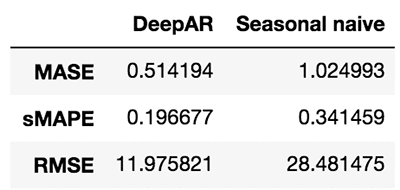## 时间序列建模任务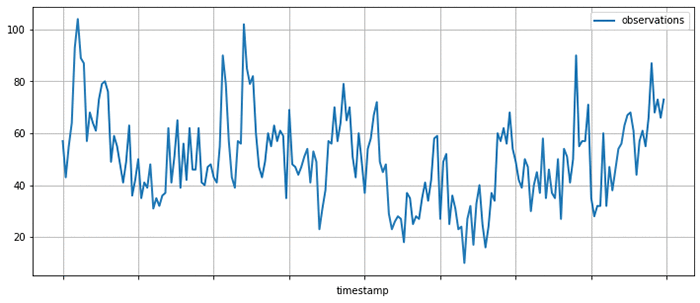• 时间序列在未来会如何变化？ ——预测
• 时间序列在某一时间段的行为是否异常？——异常检测
• 某一时间序列属于哪一个类别？——时间序列分类
• 某些未能记录到的值应该是多少？——缺失数据填充

GluonTS可以使构建时间序列模型——对于产生时间序列的过程的数学描述变得更简单，在你解决以上问题的道路上助你一臂之力。在众多时间序列模型中，GluonTS侧重于基于深度学习的模型。

## GluonTS主要功能及组件

GluonTS提供的组件可以让构造基于深度学习的时间序列模型更加简单和高效。时间序列领域的深度学习模型通常包含例如基于长-短期记忆单元的循环神经网络、卷积操作和注意力机制等模块，这些模块也存在于自然语言处理和计算机视觉等领域的模型中。由于这一点，使用Apache MXNet这样的深度学习框架来进行开发和实验是一个非常方便的做法。

• 用于构建模型的高级模块，包括诸如seq2seq这样的通用神经网络架构以及针对概率分布进行建模和转换的组件
• 时间序列数据读取和迭代器，并包含了数据转换机制
• 若干最先进的时间序列预测模型的参考实现
• 评估和比较时间序列预测模型的工具

GluonTS的大多数组件可以用于任意之前提到的时间序列建模场景，不过目前GluonTS的模型实现及周边工具还是重点着力在预测任务上。

## 使用GluonTS进行时间序列预测

• 时间序列的频率（在这里我们使用5分钟，所以我们设定freq="5min"
• 预测长度（在这里我们使用36个时间点，即3小时）

from gluonts.model.deepar import DeepAREstimator
from gluonts.trainer import Trainer
estimator = DeepAREstimator(freq="5min",
prediction_length=36,
trainer=Trainer(epochs=10))


### 在真实数据集上训练模型

import pandas as pd
import matplotlib.pyplot as plt

df[:200].plot(figsize=(12, 5), linewidth=2)
plt.grid()
plt.legend(["observations"])
plt.show()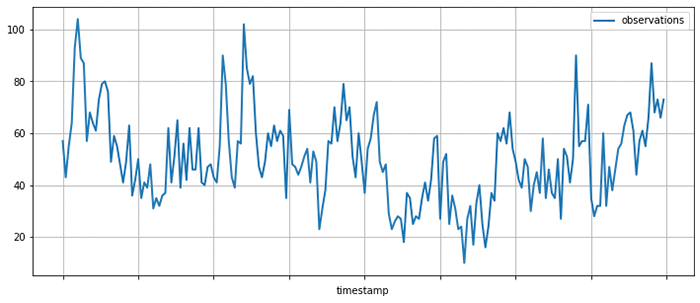GluonTS提供了Dataset抽象层来统一各种不同输入格式的读取。在这里，我们使用ListDataset来读取在内存中以字典列表形式存储的数据。在GluonTS中，任何Dataset对象都是一个将字符串键值映射到任意值的字典的迭代器。 将数据截断到2015年4月5日为止，用于训练模型。在4月5日之后的数据将被用于测试训练好的模型。

from gluonts.dataset.common import ListDataset
training_data = ListDataset(
[{"start": df.index, "target": df.value[:"2015-04-05 00:00:00"]}],
freq = "5min"
)


predictor = estimator.train(training_data=training_data)


### 模型评估

test_data = ListDataset(
[
{"start": df.index, "target": df.value[:"2015-04-10 03:00:00"]},
{"start": df.index, "target": df.value[:"2015-04-15 18:00:00"]},
{"start": df.index, "target": df.value[:"2015-04-20 12:00:00"]}
],
freq = "5min"
)


from itertools import islice
from gluonts.evaluation.backtest import make_evaluation_predictions

def plot_forecasts(tss, forecasts, past_length, num_plots):
for target, forecast in islice(zip(tss, forecasts), num_plots):
ax = target[-past_length:].plot(figsize=(12, 5), linewidth=2)
forecast.plot(color='g')
plt.grid(which='both')
plt.legend(["observations", "median prediction", "90% confidence interval", "50% confidence interval"])
plt.show()

forecast_it, ts_it = make_evaluation_predictions(test_data, predictor=predictor, num_eval_samples=100)
forecasts = list(forecast_it)
tss = list(ts_it)
plot_forecasts(tss, forecasts, past_length=150, num_plots=3)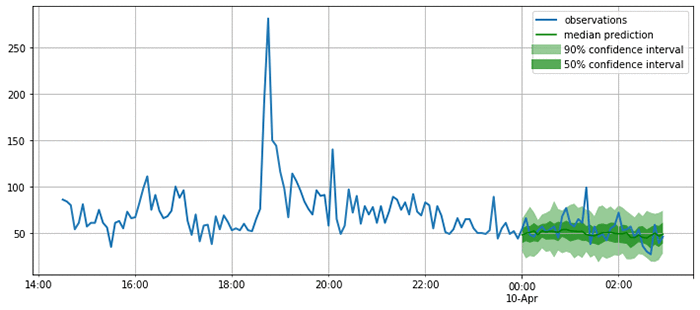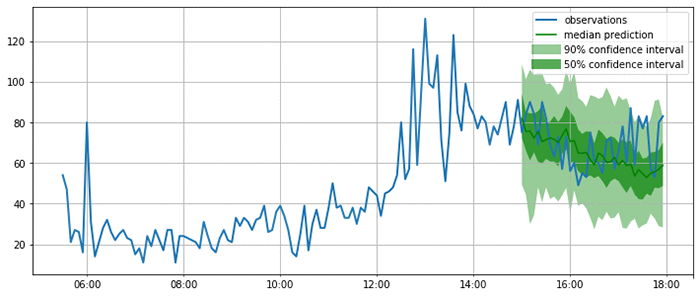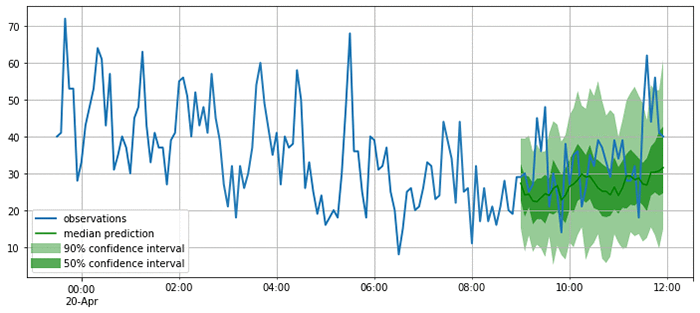from gluonts.evaluation import Evaluator
evaluator = Evaluator(quantiles=[0.5], seasonality=2016)
agg_metrics, item_metrics = evaluator(iter(tss), iter(forecasts), num_series=len(test_data))
agg_metrics
{'MSE': 163.59102376302084,
'abs_error': 1090.9220886230469,
'abs_target_sum': 5658.0,
'abs_target_mean': 52.38888888888889,
'seasonal_error': 18.833625618877182,
'MASE': 0.5361500323952336,
'sMAPE': 0.21201368270827592,
'MSIS': 21.446000940010823,
'QuantileLoss[0.5]': 1090.9221000671387,
'Coverage[0.5]': 0.34259259259259256,
'RMSE': 12.790270668090681,
'NRMSE': 0.24414090352665138,
'ND': 0.19281054942082837,
'wQuantileLoss[0.5]': 0.19281055144346743,
'mean_wQuantileLoss': 0.19281055144346743,
'MAE_Coverage': 0.15740740740740744}


from gluonts.model.seasonal_naive import SeasonalNaivePredictor

seasonal_predictor_1W = SeasonalNaivePredictor(freq="5min", prediction_length=36, season_length=2016)

forecast_it, ts_it = make_evaluation_predictions(test_data, predictor=seasonal_predictor_1W, num_eval_samples=100)
forecasts = list(forecast_it)
tss = list(ts_it)

agg_metrics_seasonal, item_metrics_seasonal = evaluator(iter(tss), iter(forecasts), num_series=len(test_data))

df_metrics = pd.DataFrame.join(
pd.DataFrame.from_dict(agg_metrics, orient='index').rename(columns={0: "DeepAR"}),
pd.DataFrame.from_dict(agg_metrics_seasonal, orient='index').rename(columns={0: "Seasonal naive"})
)

df_metrics.loc[["MASE", "sMAPE", "RMSE"]]